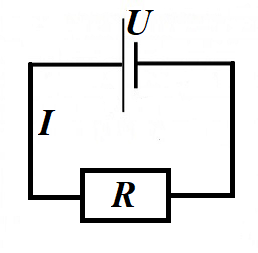## Sunday, April 19, 2020

### Unizor - Physics4Teens - Electromagnetism - Electric Heat

Notes to a video lecture on http://www.unizor.com

Direct Current - Electric Heat

Consider a circuit with a source of electricity that produces a difference in electric potential (voltage) U(volts) between its terminals. The only other component in this simple circuit is a resistor with resistance R(ohms).According to the Ohm's Law, the electric current going through a resistor is

I = U/R(amperes)

Recall that a difference in electric potential (voltage) of 1V(one volt) between two terminals of a source of electricity means that it requires one joule of work (1J) to move one coulomb of positive electric charge (+1C) between these terminals.

Therefore,

1V·1C = 1J and 1V = 1J/1C

Further recall that the electric current of 1A(one ampere) is the flow of electricity, when 1C(one coulomb) of electricity is moving across the wire within 1sec(one second).
Therefore,

1A = 1C/1sec and 1A·1sec = 1C

In case of our circuit with the voltage between the terminals of a source of electricity U, electric current I and resistor R we have I coulombs of electric charge going through a circuit each second.

So, it t seconds the source of electricity moves I·t coulombs of electricity.

Moving one coulomb (1C) of electricity between terminals with one volt (1V) of a difference in electric potential requires one joule (1J) of work.

Therefore, moving this one coulomb (1C) of electricity between terminals with a difference of electric potential (voltage) U volts requires U joules of work.

Continuing this logic, moving I·t coulombs of electricity between the terminals with the voltage U volts requires U·I·t joules of work.

So, the work performed by a source of electricity with voltage U (volts) that moves I coulombs electricity every second (amperage) through a resistor with resistance R ohms during time t sec is (in joules)

W = U·I·t

From the Ohm's Law U=I·R immediately follows

W = I²·R·t and W = U²·t / R

In most cases we will assume that wires have zero resistance and only
resistors have the property of resistance. So, in our simple circuit the
only resistance to the moving electrons is in a resistor R.

Since the source of electricity performs work, this work is supposed to
do something. According to the Law of Energy Conservation, the work is
just a transformation of one form of energy into another.

In our case the only result of the work performed by a source of
electricity is moving electrons in a circuit that, meeting resistance of
the atoms inside a resistor, push them around, thus increasing their
chaotic movement inside a resistor. This chaotic movement is heat that can be measured, for example, by measuring a temperature of a resistor.

The bottom line is that a source of electricity that has voltage V, producing an electric current with amperage I going through a resistor with resistance R during time t performs work

W = U·I·t = I²·R·t = U²·t / R

which is converted to heat that increases the temperature of a resistor.

This process is used in, for example, in incandescent lamps. The rising
temperature of a tungsten spiral inside such a lamp results in heat and
light radiation that lights and heats up the space around a lamp.